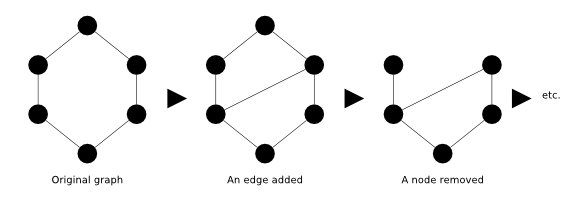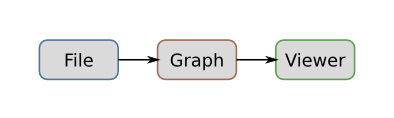# Getting Started

GraphStream is a graph handling Java library that focuses on the dynamics aspects of graphs. Its main focus is on the modeling of dynamic interaction networks of various sizes.

The goal of the library is to provide a way to represent graphs and work on it. To this end, GraphStream proposes several graph classes that allow to model directed and undirected graphs, 1-graphs or p-graphs (a.k.a. multigraphs, that are graphs that can have several edges between two nodes).

GraphStream allows to store any kind of data attribute on the graph elements: numbers, strings, or any object.

Moreover, in addition, GraphStream provides a way to handle the graph evolution in time. This means handling the way nodes and edges are added and removed, and the way data attributes may appear, disappear and evolve.In order to handle dynamic graphs, the library defines in addition to graph structures the notion of “stream of graph events”, which as you guessed, is at the origin of the library name. The number of events is restricted they are:

• node removal,
• edge removal,
• graph/node/edge attribute change,
• graph/node/edge attribute removal.
• step

Inside the library, a lot of components can generate such streams of events. These components are called sources. Other components can receive these events and process them, they are in fact very comparable to listeners, a concept widely used in the Java world. We call such components sinks.

When a component is able to both receive graph events (sink) and produce them (source) we call it a pipe. The graph structures in GraphStream are pipes. There are many kinds of pipes, that can act as filter, removing some events, or adding more events, or allowing to cross the network, or communicate between threads.

Here is an example of an often used pipeline:A reader reads graph events in a file and acts as a source, producing these events in order. Its output is connected to a graph. Each time a graph receives an event it modifies its structure to reflect the effect of the event. The graph is also a source, that is connected here to a viewer. The viewer accepts the events and shows a graphical representation of the graph.

## Installation

Before delving into code, it is important to quickly look at the project organisation in terms of library modules.

The most important and necessary one is gs-core. It defines the base graph classes, the graph event streaming classes and a minimalist, yet efficient, graph rendering user interface. The goal of this library is to remain small. This module is contained in the file `gs-core-X.Y.jar`, where `X` is the major GraphStream version, and `Y` is the minor GraphStream version. This jar is the minimal requirement.

Another important module is the gs-algo one. It provides generalist algorithms often tied to graph libraries (shortest paths, modularity, centrality, etc.) as well as what we call generators, that are algorithms that produce graphs with predefined features (random, grid, scale-free, etc.). It is defined in the `gs-algo-X-Y.jar` file.

When you download GraphStream you will find these two jars in the `lib/` directory. Simply include them in your `classpath`.

Alternatively, you can use Maven to install graphstream and setup eclipse projects as described in the maven tutorial.

## A first example

It is time to test the API. Although we emphasize on the dynamics aspects of the library, it is probably better to start with a simple static graph that we will evolve afterwards, in the following tutorials.

All graphs in GraphStream follow the `Graph` interface. You import it with:

There exist several graph implementations, a versatile one is the `SingleGraph` class. It provides a 1-graph (there can be only one edge between two nodes), that can be either directed or undirected. In fact you can mix directed and undirected edges inside such a graph, simply consider undirected edges as bidirectional. This does not well cope with the mathematical definition, but is certainly easier to use for developing. You import it with:

You create the graph this way:

There are lots of ways to populate a graph with nodes, edges and data attributes. In GraphStream the better way is to connect a graph event producer to the graph (such as a loader or graph generator). However the `Graph` class also provides a construction interface that allows to build the graph by hand. This interface is mostly useful to build small examples and to experiment with idea prototypes.

The construction API of the graph works as a factory for node and edge elements (you cannot create nodes and edges by yourself and add them in the graph, you must ask the graph to create them for you). Add the following lines after the graph declaration:

As their name suggest, these methods will add three nodes and three edges between the nodes. You can see that each node or edge is identified by a string. Such identifiers must naturally be unique.

It is often useful to check the graph by seeing it, you can easily do this using the `display()` utility method:

This should produce a result similar to this:Here is the full text of the above program:

The graph being a factory for node, it can create nodes automatically if needed. Instead of the graph construction seen above, you can use:

To produce the same result. If a node is unknown, it will be created for you.

## Exploring the graph structure

Each time you add a node or edge in the graph, a corresponding object is created for you. These objects belong to the `Node` and `Edge` interfaces. As you have seen it above, you name the nodes and edges by an identifier under the form of a string of characters. Each identifier must be unique since they relate to only one node or one edge.

Once added in a graph with an identifier, you can obtain a reference on these objects using this identifier:

or:

The `Node` and `Edge` interfaces then allow to obtain details on the represented nodes and edges. For example:

• what is the unique identifier of the node or edge (`Node.getId()`, `Edge.getId()`),
• what is the degree of the node (number of connected edges, `Node.getDegree()`),
• what are the edges connected to a node (`Node.hasEdgeToward(String id)` and `Node.getEdgeToward(String id)`),
• is an edge directed or not (`Edge.isDirected()`),
• what is the node at the other end of the edge (`Edge.getOpposite(Node n)`),
• etc.

In addition to `getNode` and `getEdge` which impose to know the identifier of the node or edge, the graph class provides ways to iterate on the nodes or edges, you can write:

The code above allows to iterate on all the nodes of the graph and will print their unique identifier, one by line. You can do a similar thing for edges:

In fact the `for(Node n:graph)` instruction is a shorthand for:

You can also obtain a read-only set of nodes from the graph (this is not a copy, but a view on the set of nodes, hence the operation is reasonably fast):

This can be useful since a lot of algorithms in the `java.util.Collections` are then applicable on the obtained set. The same operation is possible on edges using `getEdgeSet()`.

Finally, you can also, if you prefer, use iterators on theses sets of nodes and edges:

And anew, this is exactly the same for edges with `Graph.getEdgeIterator()`.